## Saturday, January 16, 2010

### Irodov Problem 3.3At any given time there are three forces acting on the spheres, i) the tension in the string T, ii) the force of gravity mg acting downwards and iii) the electrostatic repulsive force between the sphere given by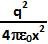. The forces responsible for the motion of the pendulum are along the tangential direction perpendicular to the string. Along this direction there are two forces acting on the sphere, i) the component of the force of gravityand ii) the component of the electrostatic force.

In order for the sphere to be in equilibrium we have,For, we have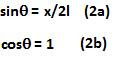From (1),(2a) and (2b) we have,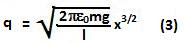As the charge slowly leaks from the spheres the electrostatic force between the spheres decreases and the equilibrium distance between the spheres decreases. Hence, we have,1.if v is changing with x, there must be some acceleration then how can we take equilibrium coditon, here?

1.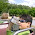We are not taking any equilibrium condition. We actually describe the acceleration by v=a/root x.

2.i did the same thing, but im still not obtaining the result.

3.If u aren't taking equilibrium then how can u substitute q2cos component equal to mgsin component.

4.in the question it should be mentioned that the charges are coming towards each other quasi statically i.e. with 0 acceleration.

5.actually this question needs some imagination and curiosity to find why charge leaks off.
This is something which i understood:-
in this question the author has written that two balls of same mass and same charge are suspended which means when they are left they attain equilibrium position and stop. now, after equilibrium has been attained, the charge starts to leak hence due to decrease in charge continuously the electrostatic force decreases and at each dt interval it attains equilibrium and this cycle of losing charge and attaining equilibrium continues.

6.actually this question needs some imagination and curiosity to find why charge leaks off.
This is something which i understood:-
in this question the author has written that two balls of same mass and same charge are suspended which means when they are left they attain equilibrium position and stop. now, after equilibrium has been attained, the charge starts to leak hence due to decrease in charge continuously the electrostatic force decreases and at each dt interval it attains equilibrium and this cycle of losing charge and attaining equilibrium continues.

7.This comment has been removed by the author.

2.the acceleration is due to net result of mgsin & qEcos component. As we have taken both of these forces in account therefore we have taken care of acceleration force automatically.

1.net resultant of qEcos and mgsin components is zero! how can that account for the acceleration then? and acceleration is not zero 'cause v is varying

3.But isn't a/sqrt.(x) velocity of approach, which will be sum of their velocities?

4.What is relation between v and x here???

1.This comment has been removed by the author.

2.5.Firstly it was given in the problem v=a/sqrt{x}, where a is a +ve constant. But the main thing is that such a velocity cannot exist. If we substitute the value of x from relation (3) here, then velocity comes which isn't the case. Moreover dQ/dt is const which is again impossible.

1.dq/dt is not a constant man! x is varying with time! only then can we have velocity! you should see the question- "v varies as a/sqrt(x) where a is a constant" which itself clarifies that x is varying......nd why should we substitute for x initially? we have to find dq/dt,not q. On differentiating we can substitute dx/dt=v=a/sqrt(x).......

2.According to the question the spheres have same charge, due to which they would repel and there would be no change in 'x' therefore there is no change in velocity and hence no acceleration

6.This comment has been removed by the author.

7.This comment has been removed by the author.

8.Velocity of approach means it should be 2(dx/dt)=v=a/{rootx}

9.why acceleration has not been taken under consideration while solving for equilibrium

10.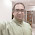here x is very less compared to l... hence it is assumed that balls approach each other slowly and at each instant for the given charge the balls are in equilibrium at their new position.

1.Hello sir, in the book the answer written is 3/2a and then the underroot part but here the answer is 3a/2 and then the underroot part now which to believe?

2.how can we assume that if x is smaller than L then speed is very slow moreover v is inversely proportional to root (x) if L be a finite common length (1m to 50m) than x is very less and velocity is very high...thus this assumption may be wrong

11.the individual velocity of each bob is u and the relative velocity of approac is v. as u is along the tangent so we have v=2ucos0 and cos0=1 so v=2u. now for solving the question we use the equilibrium but if u is constant then v should also be a constant and the question says it varies with the distance x. ???

12.hey guys in the book the answer written is 3/2a and the the underroot part but here the answer is 3a/2 and then the underroot part now which to believe?

1.actually answer written at the back is correct, it is written (3/2)a NOT (3/(a2)).

13.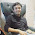equm is not to be considered. as v is fn of x we have a= v dv/dx
hence F= m a
kq^2/x^2costheta - mgsintheta= m a
hence dq/dt can be found

1.The answer won't match with the book's one that way...

14.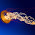Why we cant take T=mgcos0' instead of mgcos0' =T

15.Why does charge leak?

16.Why we are taking distance between mean position and 1 charge x/2 instead of x ?

17.It should be mentioned that the bodies are moving slowly, only then will the answer be justified

18.This comment has been removed by the author.

1.This comment has been removed by the author.

2.This comment has been removed by the author.

19.Nice Method. But please someone clarify that the velocity of approach is the "Relative" velocity or not..? Because then the answer will change Iff x is not so small than l.

20.plista survaaasta
,skek

21.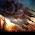What will be the answer if take the initial changes at a rate d§/DT=w.

22.What will be the answer if take the initial changes at a rate d§/DT=w.

23.All are stupids

24.Question need to improve but writer is no more .